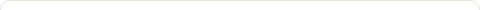# Operators in Java

Posted on: May 24, 2011

In Java we are provided with number of different operators & these operators are further divided into arithmetic, bitwise, relational and logical groups.

Arithmetic Operators

The arithmetic operators are used to perform the arithmetic operations in algebra.

 Operator Operation + Addition – Subtraction * Multiplication / Division % Modulus ++ Increment += Addition assignment -= Subtraction assignment *= Multiplication assignment /= Division assignment %= Modulus assignment — Decrement Arithmetic Operators in Java

Bitwise Operators

The bitwise operators are used to perform the different operations depending upon the individual operators.

 Operator Operation ~ Bitwise Unary NOT & Bitwise AND | Bitwise OR ^ Bitwise Exclusive OR >> Shift Right with Sign Fill >>> Shift Right with Zero Fill << Shift Left &= Bitwise AND assignment |= Bitwise OR assignment ^= Bitwise Exclusive OR assignment >>= Shift Right assignment >>>= Shift Right Zero Fill assignment <<= Shift Left assignment Bitwise Operators in Java

Relational Operators

The relational operators are used relate or to determine the relationship between the two operands.

 Operator Operation == Equal to != Not equal to > Greater than < Less than >= Greater than equal to <= Less than equal to Relational Operators in Java

Boolean Logical Operators

The boolean logical operators are used combine the two boolean operands resulting in new boolean value.

 Operator Operation & Logical AND | Logical OR ^ Logical Exclusive OR && Short-circuit AND || Short-circuit OR ! Logical Unary NOT &= AND assignment |= OR assignment ^= Exclusive OR assignment == Equal to != Not equal to ?: Ternary If-Then-Else Boolean Logical Operators in Java

The ? Operator

This is the special ternary operator in Java which is used to replace certain types of if-then-else statements.

Syntax :-

 expression1 ? expression2 : expression3

If expression1 results boolean value true then, expression2 is executed otherwise expression3 is executed.

### 2 Responses to "Operators in Java"hello there and thank you for your info – I have definitely picked up something new from right here.

LikeThanks Jones

Like